# ACU-T: 3000 Enclosed Hot Cylinder: Natural Convection

## Prerequisites

This tutorial provides instructions for setting up, solving and viewing results of a CFD simulation of air flow inside the annulus of a concentric cylinder with a heat source due to natural convection. Prior to starting this tutorial, you should have already run through the introductory HyperWorks tutorial, ACU-T: 1000 HyperWorks UI Introduction, and have a basic understanding of HyperMesh, AcuSolve, and HyperView. To run this simulation, you will need access to a licensed version of HyperMesh and AcuSolve.

Prior to running through this tutorial, copy HyperMesh_tutorial_inputs.zip from <Altair_installation_directory>\hwcfdsolvers\acusolve\win64\model_files\tutorials\AcuSolve to a local directory. Extract ACU-T3000_HotCylinder.hm from HyperMesh_tutorial_inputs.zip.

Since the HyperMesh database (.hm file) contains meshed geometry, this tutorial does not include steps related to geometry import and mesh generation.

## Problem Description

The schematic of the problem to be solved is shown in the figure above. The inner cylinder is a solid with internal heat generation and the annular space between the inner and outer cylinders is a fluid volume with air as the fluid. The air in contact with the surface of the inner cylinder is heated up and rises to the upper parts of the annulus due to buoyancy effects and displaces the cold fluid at the top. At the same time, the film of the fluid which was in contact with the hot surface is replaced by the surrounding cold fluid. This process is repeated continuously until a steady state is achieved.

Both cylinders are assumed to be infinitely long and are modeled using half symmetry and periodicity. The cylinders are infinite in z-direction and hence periodicity will be applied along this direction.

## Introduction to Theory

### Natural Convection

Convection is a heat transfer mechanism where the transfer of heat energy happens through the motion of matter. Since the definition of convection involves motion of matter a fluid state is usually present in convection. Usually this type of heat transfer takes place between a hot or a cold surface and a fluid. The film of fluid in contact with the surface absorbs heat from or transfers heat to the surface and is then replaced by a new film. This movement of fluid may either be governed by an external source, such as a fan or pump, or due to internal changes in the fluid properties. When no external sources are responsible for the fluid motion the heat transfer mechanism at work is called the Natural Convection. The driving force for motion of the fluid in a natural convection is density changes in the fluid due to temperature gradients induced in the fluid by heat transfer.

The natural convection mechanism works similarly as described above, whilst discussion of the problem. The fluid which is in contact with the surface absorbs or transfers heat from the surface and becomes hotter or colder than the surrounding fluid. Driven by buoyancy forces due to difference in densities caused by the temperature gradient, the fluid is displaced upwards or downwards. Surrounding fluid fills in the void created by the displaced fluid, which then undergoes the same process again. This gives rise to a convection current which drives the hot fluid to the top and cold fluid to the bottom of the convection cell. Buoyancy effects are driven by gravity, therefore natural convection requires presence of a gravitational force to work. It must be noted, however, that gravity is not the driving force behind the fluid movement. Presence of gravity only enables displacement of the fluid due to the density changes caused by temperature gradients.

Mathematical determination of the onset of natural convection is done through a dimensionless number called the Rayleigh number (Ra). The Rayleigh number is defined as:

where:
• x is the characteristic length (m)
• $R{a}_{x}$ is the Rayleigh number for characteristic length x
• $g$ is acceleration due to gravity (m/s2)
• ${T}_{s}$ is the surface temperature (K)
• ${T}_{\infty }$ is the quiescent temperature (fluid temperature far from the surface of the object) (K)
• $\nu$ is the kinematic viscosity (m2/s)
• α is the thermal diffusivity (m2/s)
• β is the thermal expansion coefficient (equals to $1/T$ for ideal gases where is absolute temperature).

The fluid properties $\nu$, α and β are evaluated at the film temperature, ${T}_{f}$, which is defined as:

${T}_{f}=\frac{{T}_{s}+{T}_{\infty }}{2}$

When the Rayleigh number is below a critical value for the fluid heat transfer is primarily in the form of conduction. When it exceeds this critical value the dominant heat transfer mechanism is convection.

### Boussinesq Density Model

The Boussinesq density model is an approximation method applied to buoyancy driven flows, such as natural convection flows. In the Boussinesq approximation, the density variation terms are neglected everywhere except when multiplied by acceleration due to gravity, $g$. The basis of this approximation is that since temperature changes are small, the resultant changes in density are small as well and thus can be neglected. However, when multiplied by $g$, the resultant term gives rise to forces which no longer are negligible. The Boussinesq approximation is:

where
• $\rho$ is the instantaneous density at temperature $T$ (kg/m3)
• ${\rho }_{0}$ is the density at reference temperature ${T}_{o}$ (kg/m3)
• $\text{Δ}T$ is change in temperature $T-{T}_{o}$ (K)

As stated in the approximation, the Boussinesq density model is only applicable when density variations are small. A general guideline is to check for the condition to be true. This indirectly puts a limitation on this model to be used to only for cases where expected temperature differences within the fluid are not large.

## Open the HyperMesh Model Database

1. Start HyperMesh and load the AcuSolve user profile.
Refer to the HM introductory tutorial, ACU-T: 1000 HyperWorks UI Introduction, to learn how to select AcuSolve from User Profiles.
2. Click the Open Model icon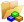located on the standard toolbar.
The Open Model dialog opens.
3. Browse to the directory where you saved the model file. Select the HyperMesh file ACU-T3000_HotCylinder.hm and click Open.
4. Click File > Save As.
The Save Model As dialog opens.
5. Create a new directory named NaturalConvection and navigate into this directory.
This will be the working directory and all the files related to the simulation will be stored in this location.
6. Enter NaturalConvection as the file name for the database, or choose any name of your preference.
7. Click Save to create the database.

## Set the General Simulation Parameters

In this step, you will set the simulation parameters that apply globally to the simulation.

### Set the General Simulation Parameters

1. Go to the Solver Browser, expand 01.Global, then click PROBLEM_DESCRIPTION.
2. In the Entity Editor, verify that the Analysis type is set to Steady State.
3. Set the Temperature equation to Advective Diffusive.

### Specify the Solver Settings

1. In the Solver Browser, click 02.SOLVER_SETTINGS under 01.Global.
2. In the Entity Editor, change the Convergence tolerance to 0.0001.
3. Verify that the Flow and Temperature fields are turned On.
4. Turn On Temperature flow.

### Create Time History Output Points

The Time History Output command enables you to extract the nodal solution at any point within the domain.

1. In the Solver Browser, right-click on 18.Time_History_Output and select Create.
2. In the Entity Editor, rename the output as Monitor Points.
3. Change the Type to Coordinates.
4. Set the No of Coordinate Points to 2.
A new data entry field is created.
5. Click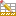to open the No of Coordinates dialog. In the dialog, enter the values as shown in the figure below then close the dialog.
6. Set the Output frequency to 1.
By setting this, the surface output is written for each time step at the specified coordinate points.

## Set the Body Force and Material Model Parameters

### Modify the Material Model Parameters of Air

1. In the Solver Browser, expand 02.Materials > FLUID then click on Air_HM.
2. In the Entity Editor, change the Density type to Boussinesq.
3. Verify that the Expansivity and Reference temperature values are set to 0.00347222222222 K-1 and 288 K respectively.

### Create a Material Model for the Solid

1. In the Solver Browser, right-click on 02.Materials and select Material(Solid).
2. In the Entity Editor, rename the material as Stainless Steel.
3. Set the Density value to 8000 kg/m3.
4. Set the Specific heat value to 500 J/kg-K.
5. Set the Conductivity value to 16.2 W/m-K.

### Define the Gravitational Body Force

1. In the Solver Browser, expand 03.Body_Force > BODY_FORCE then click on Gravity_HM.
2. In the Entity Editor, change the value of Y Gravity to -9.81 ,/sec2.
3. Change the Z Gravity to 0.

### Define the Volumetric Heat Source

1. In the Solver Browser, right-click on 03.Body_Force and select Create.
2. In the Entity Editor, rename the body force as Heat Source.
3. Change the Medium to Solid.
4. Change the Heat source unit type to Per unit volume.
5. Set the Volumetric heat source value to 20000 W/m3.
6. Save the model.

## Specify the Boundary Conditions and Initial Conditions

### Specify the Material Properties and Surface Boundary Conditions

By default, all components are assigned to the wall boundary condition. In this step, you will change them to the appropriate boundary conditions and assign material properties to the fluid volumes.
1. In the Solver Browser, expand 12.Surfaces > WALL.
2. Click Solid. In the Entity Editor,
1. Change the Type to SOLID.
2. Select Stainless Steel as the Material.
3. Set the Body force to Heat Source.
3. Click Fluid. In the Entity Editor,
1. Change the Type to FLUID.
2. Select Air_HM as the Material.
3. Set the Body force to Gravity_HM.
4. Click solid_pos_z. In the Entity Editor, turn Off the simple boundary condition.
5. Similarly, turn off the simple boundary condition for the solid_neg_z, fluid_pos_z and fluid_neg_z components.
These surfaces are the periodic surfaces along the length of the domain and should be modeled as internal surfaces. Hence, the simple boundary condition can be turned off.
6. Click symmetry. In the Entity Editor, change the Type to SYMMETRY.
7. Click inner_wall. In the Entity Editor, verify that the Type is set to WALL.
8. Click outer_wall. In the Entity Editor,
1. Verify that the Type is set to WALL.
2. Change the Temperature BC type to Value.
3. Set the Temperature to 298 K.

### Specify the Periodic Boundary Conditions

Since you are modeling only a portion of the infinitely long domain, you need to define the periodicity of the solution by specifying periodic boundary conditions. The solution is then constrained by periodicity. The periodic boundary condition links the corresponding pairs of nodes on the two surfaces on which the periodicity is defined.

1. In the Solver Browser, right-click on 16.Periodic_Boundary_Condition and select Create.
2. In the Entity Editor, rename the boundary condition as periodicity_fluid.
3. Verify that the Definition is set to Periodic components.
4. In the Source component field, click the Component entity collector and select fluid_pos_z from the list of components.
5. Similarly, set the Target component to fluid_neg_z.
6. Repeat steps 1 through 5 and create another PBC named periodicity_solid with the Source and Target components as solid_pos_z and solid_neg_z, respectively.

### Specify the Reference Pressure

Since this model set up doesn’t have an inlet and outlet, we need to specify the reference pressure manually. This can be done by specifying a pressure nodal boundary condition at a node in the fluid region.

1. In the Solver Browser, right-click on 15.Nodal_Boundary_Condition and select Create.
2. In the Entity Editor, rename the boundary condition as Fixed Pressure node.
3. Change the Definition to Nodes.
4. Set the Number of Nodes to 1.
5. Change the Boundary condition variable to Pressure.
6. Verify that the Type is set to Zero.
7. Click the Node entity collector then pick a node in the fluid region from the modeling window.

### Specify the Nodal Initial Conditions

1. In the Solver Browser, expand 01.Global then click on NODAL_INITIAL_CONDITION.
2. In the Entity Editor, set the Default value for Temperature to 353.15 K.
3. Save the model.

## Compute the Solution

1. Turn on the visibility of all mesh components.
For the analysis to run, the mesh for all active components must be visible.
2. Click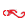on the ACU toolbar.
The Solver job Launcher dialog opens.
3. Optional: For a faster solution time, set the number of processors to a higher number (4 or 8) based on availability.
4. The Output time steps can be set to All or Final. Since this is a steady state analysis, the Final time step output is sufficient.
5. Make sure that the Auto run AcuTail and AcuProbe options are turned On.
6. Leave the remaining options as default and click Launch to start the solution process.

## Post-Process the Results

As the solution progresses, the AcuProbe and AcuTail windows are launched automatically. The progress of the solution can be monitored using these applications.

### Post-Process with AcuProbe

1. Once the solution has converged, from the AcuProbe Data Tree, expand Time History > Monitor Points > node 1.
2. Right-click on temperature and select Plot.
3. Similarly, expand node 2, right-click on temperature, and select Plot.
The temperature plot at both the locations should be displayed in the plot window as shown below.
Note: You might need to click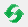on the toolbar in order to properly display the plot.
Close the AcuTail and AcuProbe windows. In the HyperMesh window, close the AcuSolve Control tab.

### Open HyperView and Load the Model and Results

1. In the HyperMesh main menu area, click Applications > HyperView.
Once the HyperView window is loaded, the Load model and results panel should be open by default. If you do not see the panel, click File > Open > Model.
2. In the Load model and results panel, click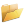next to Load model.
3. In the Load Model File dialog, navigate to your working directory and select the AcuSolve .Log file for the solution run that you want to post-process. In this example, the file to be selected is NaturalConvection.1.Log.
4. Click Open.
5. Click Apply in the panel area to load the model and results.
The model is colored by geometry after loading.

### Create a Temperature Distribution Contour

1. Click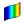on the Results toolbar to open the Contour panel.
2. In the panel area, change the Result type to Temperature.
3. Click Apply to display the temperature distribution contour.
4. Orient the display to the xy-plane by clicking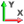on the Standard Views toolbar.
5. In the panel area, under the Display tab, turn off the Discrete color option.
6. Click the Legend tab then click Edit Legend. In the dialog, change the Numeric format to Fixed then click OK.
Verify that the contour plot looks like the figure shown below.

### Create a Velocity Vector Plot

1. Click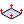on the Results toolbar to open the Vector panel.
2. In the panel area, verify that the Result type is set to Velocity(v) and X+Y+Z is the only check box activated.
3. Activate the Overlap result display check box.
This will imprint the vector plot on top of the temperature contour plot created in the previous step.
4. Click Apply in the panel area.
5. Go to the Display tab.
6. Change the Color by option to Direction.
7. Click the color button beside X+Y_Z and set it to black (or any color of your preference).
8. Set the Vector heads options to Arrow and Tip.

The Vector plot should be modified accordingly as you make the changes mentioned above. Verify that the vector plot looks like the one shown in the figure below. The plot is zoomed in for a clear display of the direction of air flow.

As mentioned in the problem description, the air which is in contact with the solid surface gets heated up and rises to the top while being replaced by the colder air and the cycle gets repeated until steady state is reached.

## Summary

In this tutorial, you successfully learned how to set up and solve a natural convection problem using AcuSolve in HyperMesh. You started by importing the HyperMesh model database and then set up the simulation parameters and boundary conditions. Once the solution was computed, you post-processed the results using HyperView and created a contour plot of temperature distribution across the domain.